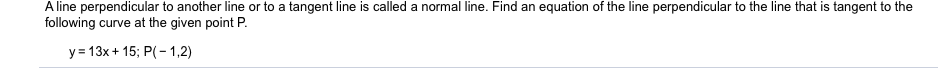# A line perpendicular to another line or to a tangent line is called a normal line. Find an equation of the line perpendicular to the line that is tangent to thefollowing curve at the given point Py 13x+ 15; P(-1,2)

Question
1 viewshelp_outlineImage TranscriptioncloseA line perpendicular to another line or to a tangent line is called a normal line. Find an equation of the line perpendicular to the line that is tangent to the following curve at the given point P y 13x+ 15; P(-1,2) fullscreen
check_circle

Step 1

Slope of the normal ...

### Want to see the full answer?

See Solution

#### Want to see this answer and more?

Solutions are written by subject experts who are available 24/7. Questions are typically answered within 1 hour.*

See Solution
*Response times may vary by subject and question.
Tagged in

### Derivative